# Understanding different Box Plot with visualization

Let’s see how can boxplot be useful in different ways.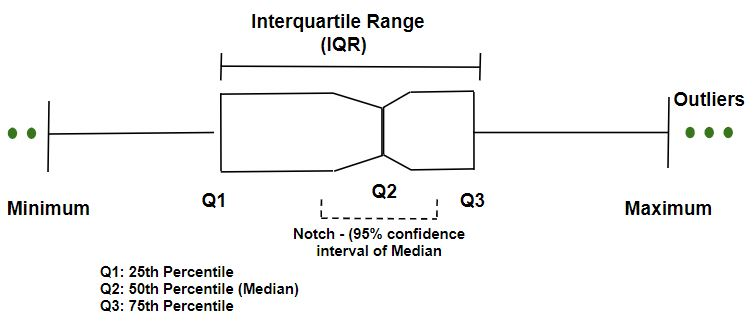`import` `numpy as np ` `import` `pandas as pd ` `import` `matplotlib.pyplot as plt `

Preparing Data

 `spread ``=` `np.random.rand(``50``) ``*` `100` `center ``=` `np.ones(``25``) ``*` `50` `flier_high ``=` `np.random.rand(``10``) ``*` `100` `+` `100` `flier_low ``=` `np.random.rand(``10``) ``*` `-``100` `data ``=` `np.concatenate((spread, center, flier_high, flier_low), ``0``) ` ` `  `print` `(data) `

Output :

```[ 35.94741387  98.49500418  37.2487085   93.19618571   6.34263359
49.10532713  53.86860981  58.59362227  36.96325746  62.27757508
65.44118887  73.79592156  95.15399991  79.94114982  16.64273792
88.35737021  14.84581489   0.76759854  91.61486239  16.03299406
73.12589808   8.63636833  33.25606049  46.05712779  81.60993207
95.0390852   43.94169286   2.96961334  38.21446718  12.15763603
8.79716665  61.18542821  70.93695599  48.90136391  54.6233727
77.27315695  14.63597135  68.22763576  52.23548596  14.34491407
55.53669512  93.63144771  15.66242535  72.47360029  67.82493039
0.34568417  63.39884046   0.46750944  70.39370656  83.42420235
50.          50.          50.          50.          50.
50.          50.          50.          50.          50.
50.          50.          50.          50.          50.
50.          50.          50.          50.          50.
50.          50.          50.          50.          50.
134.61039367 133.42423423 132.77938791 157.75858139 105.99552891
159.1713425  190.9938417  118.33354777 142.13310114 113.54291724
-32.73427425 -34.92884623 -49.28116565 -15.24891626 -14.57460618
-9.48256045 -46.74250253 -36.3992666  -88.14980994 -64.49187441]```

Code #1: Normal Box Plot

 `plt.figure(figsize ``=` `(``7``, ``5``)) ` `plt.boxplot(data) ` ` `  `plt.show() `

Output :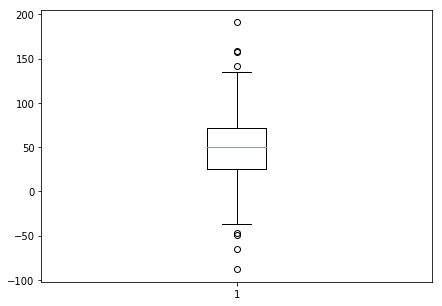Code #2: Notch Box Plot

 `plt.figure(figsize ``=` `(``7``, ``5``)) ` `plt.boxplot(data, ``1``) ` ` `  `plt.show() `

Output :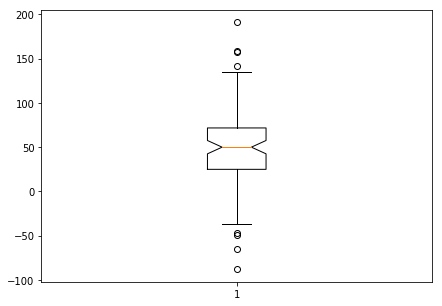Code #3: Box Plot showing Outliers

 `plt.figure(figsize ``=` `(``7``, ``5``)) ` `plt.boxplot(data, ``0``, ``'gD'``) ` ` `  `plt.show() `

Output :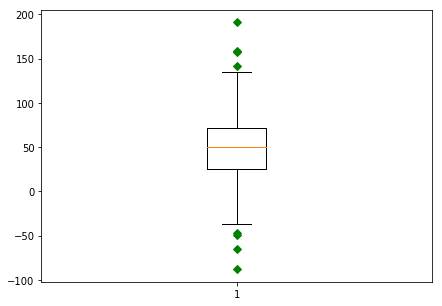Code #4: Box Plot without Outliers

 `plt.figure(figsize ``=` `(``7``, ``5``)) ` `plt.boxplot(data, ``0``, '') ` ` `  `plt.show() `

Output :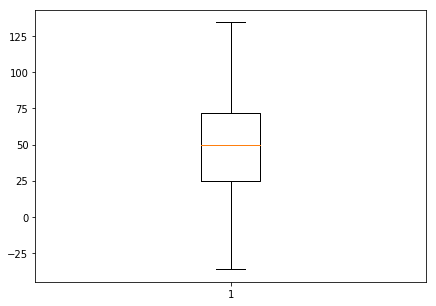Code #5: Horizontal Box Plot

 `plt.figure(figsize ``=` `(``7``, ``5``)) ` `plt.boxplot(data, ``0``, ``'rs'``, ``0``) ` ` `  `plt.show() `

Output :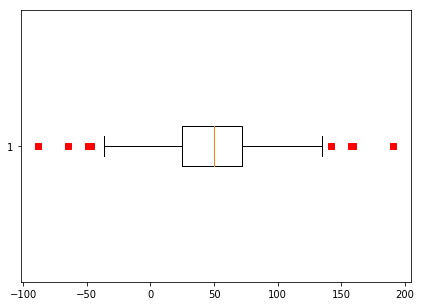Code #6: Horizontal Box Plot changing Whiskers length

 `plt.figure(figsize ``=` `(``7``, ``5``)) ` `plt.boxplot(data, ``0``, ``'rs'``, ``0``, ``0.75``) ` ` `  `plt.show() `

Output :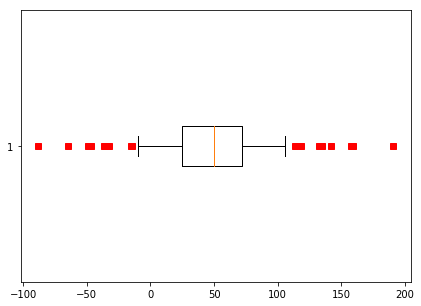Attention geek! Strengthen your foundations with the Python Programming Foundation Course and learn the basics.

To begin with, your interview preparations Enhance your Data Structures concepts with the Python DS Course.

My Personal Notes arrow_drop_upAspire to Inspire before I expire

If you like GeeksforGeeks and would like to contribute, you can also write an article using contribute.geeksforgeeks.org or mail your article to contribute@geeksforgeeks.org. See your article appearing on the GeeksforGeeks main page and help other Geeks.

Please Improve this article if you find anything incorrect by clicking on the "Improve Article" button below.

Improved By : ManasChhabra2, nidhi_biet

Article Tags :
Practice Tags :

1

Please write to us at contribute@geeksforgeeks.org to report any issue with the above content.